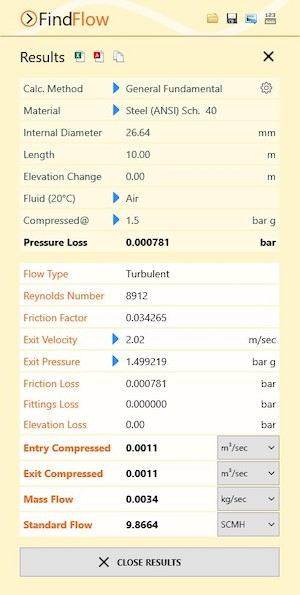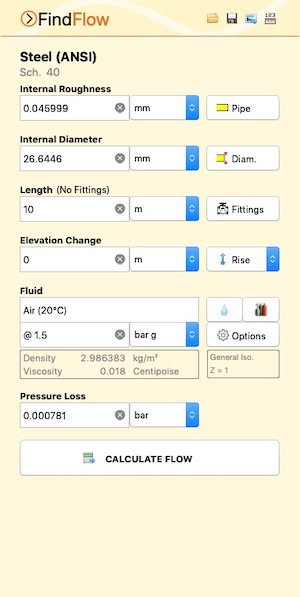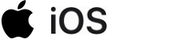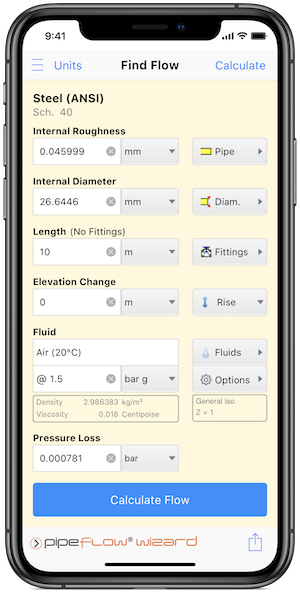# Pipe Flow Wizard Software - Flow Rate Calculator

The Pipe Flow Wizard Software will calculate the flow rate in a pipe that occurs for a given pressure difference between the start of the pipe and end of the pipe (the available pressure difference). The software will consider the size of the internal pipe diameter, the internal roughness of the pipe material, the length of pipe, the friction loss in the pipe for the flow velocity, and the pressure loss through pipe fittings & bends.

## Liquids and Gases

The Pipe Flow Wizard software uses the Darcy Weisbach equation with accurate Colebrook-White friction factors when calculating friction losses and overall pressure drop in the pipe for liquids.

The Pipe Flow Wizard software uses Compressible Isothermal Flow equations when calculating the friction losses and total pressure drop in the pipe for gases. The software supports the General Fundamental Flow equation, Complete Isothermal Flow equation, AGA Flow equation, Weymouth Flow equation, Panhandle A Flow equation, Panhandle B Flow equation, and the IGT Flow equation.

## Pipes and Fittings

The Pipe Flow Wizard software comes with standard data (which can be added to) that allows the user to easily select pipe material, pipe diameter, pipe fittings and the fluid to be used in the calculation.Find Flow calculation and results screenshots from Pipe Flow Wizard Software for Windows.Find Flow Calculation Screen (Windows)Find Flow Results Screen (Windows)Find Flow calculation and results screenshots from Pipe Flow Wizard Software for macOS.Find Flow Calculation Screen (macOS)Find Flow Results Screen (macOS)Find Flow calculation and results screenshots from Pipe Flow Wizard Software for iOS.Find Flow Calculation Screen (iOS)
Find Flow Results Screen (iOS)

Secure Online PaymentsPipe Flow Software: Piping design, Pressure drop calculator, Flow rate calculator, Pump head calculations, Pump selection software.  Copyright © Pipe Flow Software 1997-2020